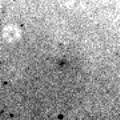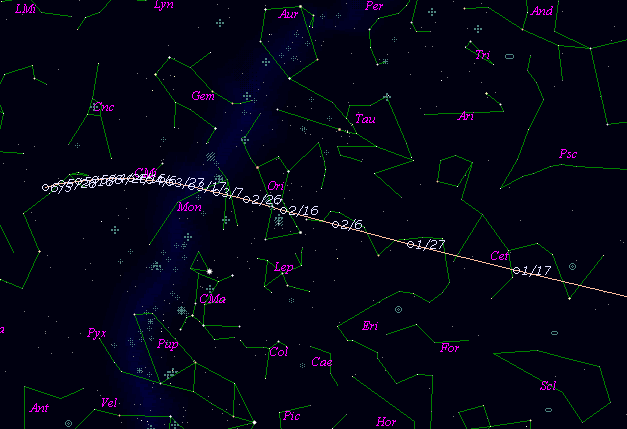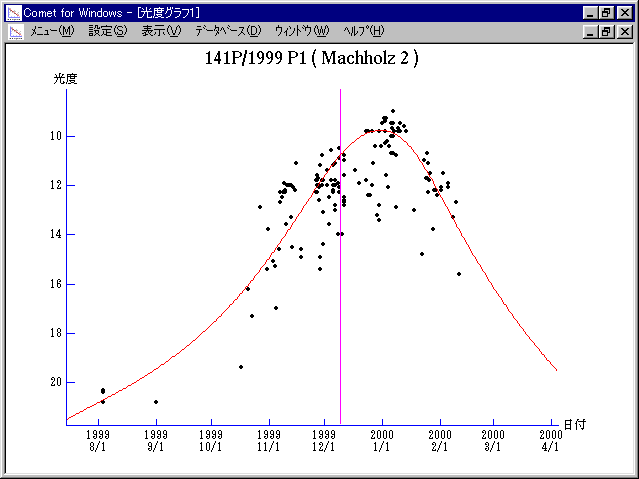# \$B%^%C%/%[%k%DBh#2WB@1(B

141P/Machholz 2 (1999)

 English version Home page Updated on September 29, 2004###\$B%W%m%U%#!<%k(B

 \$BId9f(B 141P/1999 P1 \$B8!=PF|(B 1999\$BG/(B8\$B7n(B3\$BF|(B \$B8!=P8wEY(B 20.8\$BEy(B \$B8!=P R. H. McNaught

###\$B###\$B50F;MWAG(B

``` Comet 141P/Machholz 2 ( Nucleus A )

The following improved orbital elements by Kenji Muraoka, are
from 234 observations  1994 to 2000, including 9 Planets, Moon
and 5 minor planets perturbations and non-gravitational effect
of style II.   The mean residual is +/- 0.83 arc seconds.

Epoch  =  1999 Dec.  8.0  TT       JDT = 2451520.5
T  =  1999 Dec.  9.27438       +/- 0.00006 (m.e.) TT
Peri. =  149.30205                +/- 0.00018
Node  =  246.13618                +/- 0.00013   (2000.0)
Incl. =   12.81126                +/- 0.00003
q  =    0.7489322              +/- 0.0000005 AU
e  =    0.7510566              +/- 0.0000006
a  =    3.0084442              +/- 0.0000025 AU
n  =    0.18888225             +/- 0.00000024
P  =    5.218                  +/- 0.0000065  years
A1  =   +0.504                  +/- 0.020
A2  =   -0.19475                +/- 0.00325

Comet 141P/Machholz 2 ( Nucleus D )

The following improved orbital elements by Kenji Muraoka,
are from 114 observations 1994 to 1999, including 9 Planets,
Moon and 5 minor planets perturbations and non-gravitational
effect of style II.  The mean residual is +/- 1.09 arc seconds.

Epoch  =  1999 Dec.  8.0  TT       JDT = 2451520.5
T  =  1999 Dec.  9.95662       +/- 0.00025 (m.e.) TT
Peri. =  149.29339                +/- 0.00088
Node  =  246.13491                +/- 0.00040   (2000.0)
Incl. =   12.81195                +/- 0.00012
q  =    0.7489913              +/- 0.0000037 AU
e  =    0.7510778              +/- 0.0000081
a  =    3.0089376              +/- 0.0000331 AU
n  =    0.18883579             +/- 0.00000311
P  =    5.219                  +/- 0.0000861  years
A1  =   +1.605                  +/- 0.146
A2  =   +0.42056                +/- 0.04229
```

###\$B@1?^(B###\$B8wEYJQ2=(B

```        m1 = 14 + 5 log\$B&\$(B + 20 log r(t - 10)

A\$B3K!'(B
m1 = 14 + 5 log\$B&\$(B + 20 log r(t - 10)

D\$B3K!'(B
m1 = 18.5 + 5 log\$B&\$(B + 55 log r(t + 20)
```##### \$B50F;MWAG\$OB<2,7r<#;a\$N7W;;\$K\$h\$k\$b\$N\$G\$9!#(B \$B@1?^\$O(B StellaNavigator Ver.2.0 for Windows (\$B%"%9%H%m%"!<%D(B \$BJTCx(B / \$B%"%9%-!<=PHG6I4)(B) \$B\$G:n@.\$7\$?\$b\$N\$G\$9!#(B \$B8wEY%0%i%U\$O(BComet for Windows\$B\$G:n@.\$7\$?\$b\$N\$G\$9!#(B